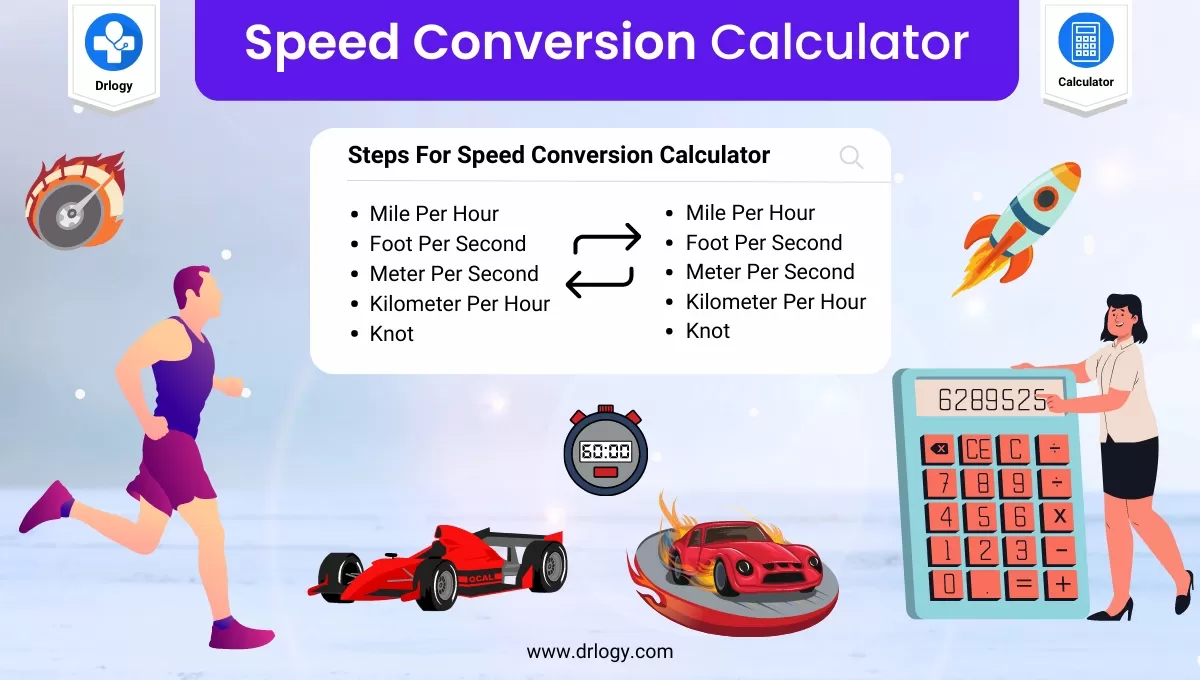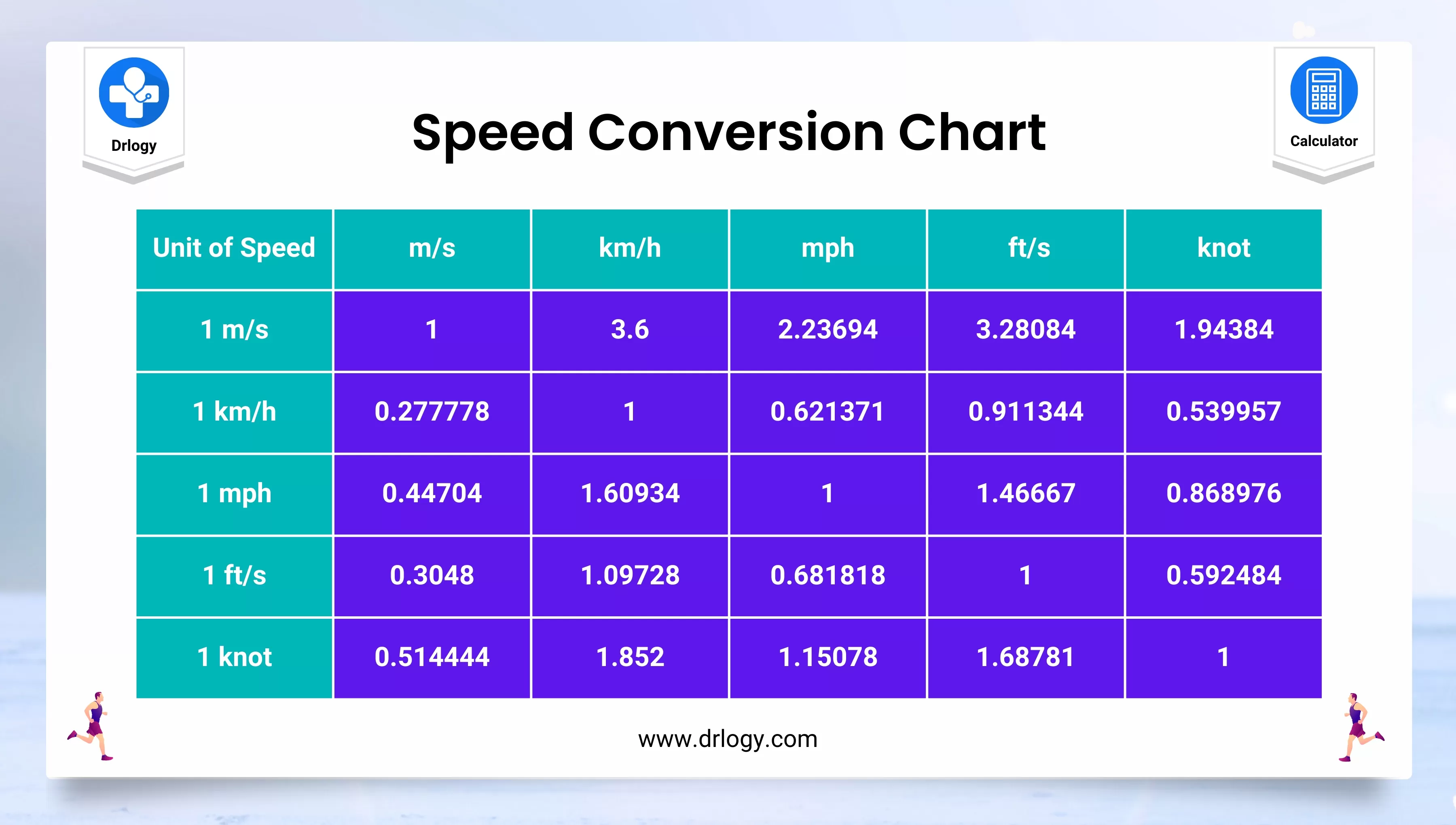• Health
• Pet
• Unit Conversion
• Medical# Speed Conversion Calculator - Speed Units Converter

## Speed Conversion Calculator

Result : =
keyboard_arrow_down
=
keyboard_arrow_down

## Speed Conversion Calculator

• Speed conversion calculator is an online tool that allows users to convert different units of speed.
• The speed conversion calculator is designed to help users easily convert speeds from one unit to another, such as miles per hour to kilometers per hour or meters per second to feet per minute.
• With a speed conversion calculator, users can quickly and accurately convert speeds for various purposes, such as for use in scientific calculations, engineering projects, or simply for everyday use.
• The speed conversion calculator is particularly useful for individuals who work with different speed units or who need to make quick calculations for speed conversions.

## Steps For Speed Conversion Calculator

Here are the steps to use a speed conversion calculator:

1. Identify the units of speed that you want to convert from and to. Most conversion calculators will provide a drop-down menu or list of options to select from.
2. Enter the speed value that you want to convert into the appropriate field. Make sure that you enter the value in the correct units.
3. The result will be displayed in the corresponding field or box.
4. If you need to perform additional conversions, simply repeat steps 2-4 as needed.
5. If the calculator has the option, you can also select the number of decimal places that you want the result to be rounded to.
6. Some calculators may also have the option to reverse the conversion, allowing you to convert back to the original units.
7. Once you have completed your conversions, you can close the calculator or clear the fields to start a new set of conversions.

Overall, using a speed conversion calculator is a quick and easy way to convert between different units of speed without having to perform manual calculations or look up conversion factors.

## Speed Conversion Chart

Here is a speed conversion chart that includes some of the most commonly used units of speed:

Unit of Speed Symbol Conversion Factor
Meters per second m/s 1
Kilometers per hour km/h 0.2777778
Miles per hour mph 0.44704
Knots kn 0.5148
Feet per second ft/s 0.3048
• To use this chart, simply find the unit of speed you want to convert from and then multiply or divide by the conversion factor to get the equivalent value in another unit.
• For example, to convert 50 mph to km/h, you would multiply 50 by 1.60934 (the conversion factor from miles per hour to kilometers per hour), giving you a result of 80.467 km/h.## Speed Converter Units or Parameters

Here are the units or parameters for the speed converter.

• Mile Per Hour
• Foot Per Second
• Meter Per Second
• Kilometer Per Hour
• Knot

## Speed Conversion Formulas

Here are some common speed conversion formulas for converting between different units of speed:

1. Meters per second to kilometers per hour: km/h = m/s x 3.6
2. Kilometers per hour to meters per second: m/s = km/h ÷ 3.6
3. Miles per hour to kilometers per hour: km/h = mph x 1.60934
4. Kilometers per hour to miles per hour: mph = km/h ÷ 1.60934
5. Feet per second to meters per second: m/s = ft/s x 0.3048
6. Meters per second to feet per second: ft/s = m/s ÷ 0.3048
7. Knots to kilometers per hour: km/h = knots x 1.852
8. Kilometers per hour to knots: knots = km/h ÷ 1.852

These formulas are based on the conversion factors between the units of speed. To use them, simply plug in the value that you want to convert and perform the calculation.

## What is Speed

• Speed is a measure of how fast an object is moving. It is defined as the distance traveled by an object in a certain amount of time.
• In physics, speed is typically measured in units of meters per second (m/s) or kilometers per hour (km/h), but it can also be expressed in other units such as miles per hour (mph), feet per second (ft/s), or knots.
• The speed of an object can be calculated by dividing the distance traveled by the time it takes to travel that distance. For example, if a car travels 100 kilometers in 2 hours, its speed can be calculated as:

Speed = Distance ÷ Time Speed = 100 km ÷ 2 hours Speed = 50 km/h

• Speed is a scalar quantity, which means that it only has magnitude (how fast an object is moving) but no direction.
• In contrast, velocity is a vector quantity that includes both speed and direction.
• While speed tells you how fast an object is moving, velocity tells you how fast it is moving and in what direction.

## How to Convert Units of Speed

To convert units of speed, follow these general steps:

1. Identify the units of speed that you want to convert from and to. Common units of speed include meters per second (m/s), kilometers per hour (km/h), miles per hour (mph), feet per second (ft/s), and knots.
2. Find the conversion factor between the two units of speed. You can usually find these conversion factors online or in a conversion table. For example, to convert kilometers per hour to miles per hour, the conversion factor is 0.621371.

Multiply the value that you want to convert by the conversion factor. For example, if you want to convert 60 km/h to mph:

60 km/h x 0.621371 = 37.2828 mph

• Round the converted value to the desired number of decimal places, if necessary.
• Write the converted speed value with the appropriate units.
• Note that when converting between different units of speed, it is important to pay attention to the direction of the conversion.
• For example, to convert from km/h to mph, you would multiply by a conversion factor that is less than 1, while to convert from mph to km/h, you would multiply by a conversion factor that is greater than 1.

## Speed Conversion Calculator Benefits

A speed conversion calculator can be a useful tool in a variety of settings. Here are some benefits of using a speed conversion calculator:

1. Saves Time: Calculating speed conversions manually can be time-consuming, especially if you need to convert between different units frequently. Using a calculator can save you time and effort.
2. Accurate Results: Speed conversion calculators are designed to provide accurate results, eliminating the risk of errors that may occur when calculating manually.
3. Easy to Use: Speed conversion calculators are usually easy to use, even for people who are not familiar with the formulas involved in speed conversion.
4. Multifunctional: Speed conversion calculators can handle conversions between different units of speed, such as meters per second, miles per hour, knots, and more.
5. Useful in Different Fields: A speed conversion calculator can be used in a variety of fields, including transportation, sports, engineering, physics, and more.
6. Helps to Make Decisions: Knowing the speed of an object or vehicle can help make informed decisions, such as determining the time needed to reach a destination or calculating fuel consumption.

Summary

Overall, a speed conversion calculator is a valuable tool that can help simplify and streamline speed conversions in various settings. Check more unit conversion calculators like this to solve your daily problems on Drlogy Calculator to get exact solution.

Reference

• Template Speed conversions Wikipedia .
• Speed, Acceleration, and Time Unit Conversions .
favorite_border 9856 Likes

## Speed Conversion Calculator FAQ

### How do you convert km to speed?

Kilometers (km) is a unit of distance, not speed. However, you can convert kilometers per hour (km/h) to other units of speed. Here's how to convert km/h to some other common units of speed:

1. To convert km/h to meters per second (m/s), divide the value in km/h by 3.6. For example, 60 km/h is equivalent to 16.67 m/s (60 ÷ 3.6 = 16.67).

2. To convert km/h to miles per hour (mph), multiply the value in km/h by 0.6214. For example, 60 km/h is equivalent to 37.28 mph (60 × 0.6214 = 37.28).

3. To convert km/h to feet per second (ft/s), multiply the value in km/h by 0.9114. For example, 60 km/h is equivalent to 54.68 ft/s (60 × 0.9114 = 54.68).

These formulas can be used to convert any value in kilometers per hour to the desired unit of speed.

### How do you calculate speed in meters per second?

• To calculate speed in meters per second (m/s), you need to know the distance traveled in meters and the time taken in seconds. The formula for calculating speed is:

Speed = distance ÷ time

• For example, let's say you run a distance of 200 meters and it takes you 25 seconds. To calculate your speed in meters per second, you would use the formula as follows:

Speed = distance ÷ time speed = 200 meters ÷ 25 seconds speed = 8 meters per second

• Therefore, your speed would be 8 m/s. It's important to make sure that the units of distance and time are consistent, so if the distance is in kilometers and the time is in minutes, you would need to convert one or both units to meters and seconds before using the formula.

### What is the formula for converting km hr to m s?

• To convert kilometers per hour (km/h) to meters per second (m/s), you can use the following formula:

m/s = km/h ÷ 3.6

• This formula works because there are 3.6 seconds in one hour (3600 seconds ÷ 1 hour), so to convert kilometers per hour to meters per second, you need to divide the value in kilometers per hour by 3.6.
• For example, let's say you are traveling at a speed of 60 km/h. To convert this speed to meters per second, you would use the formula as follows:

m/s = km/h ÷ 3.6 m/s = 60 ÷ 3.6 m/s = 16.67

• Therefore, 60 km/h is equivalent to 16.67 m/s.

View More Health

## Medical Calculator

View More Medical

View More Pet

## Unit Conversion Calculator

View More Unit Conversion

## Financial Calculator

View More Financial
• Home >
• Calculator >
• Speed Conversion Calculator - Speed Units Converter
Drlogy®
The Power To Health